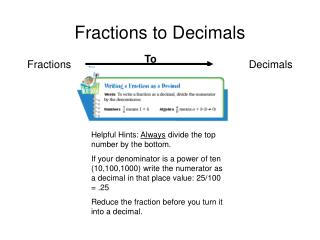DownloadDownload PresentationFractions to Decimals

# Fractions to Decimals

Télécharger la présentation## Fractions to Decimals

- - - - - - - - - - - - - - - - - - - - - - - - - - - E N D - - - - - - - - - - - - - - - - - - - - - - - - - - -
##### Presentation Transcript

1. Fractions to Decimals To Fractions Decimals Helpful Hints: Always divide the top number by the bottom. If your denominator is a power of ten (10,100,1000) write the numerator as a decimal in that place value: 25/100 = .25 Reduce the fraction before you turn it into a decimal.

2. 4 3 Fractions to Decimals Mixed Numbers to decimals: Types of Decimals: Terminating: Repeating: Truncate:

3. Using Division: A fraction is a division problem. ¾ means: 3 ÷ 4. Fractions and decimals represent the same value in different forms. ½ = 0.5. ⌐ 4 3 Hint: The numerator or (top number) always goes inside the house Fractions to Decimals Mixed Numbers to decimals: 3 3/4 Keep the whole number the same. Change the fraction to a decimal. Put the whole number with the decimal. 3. 75 Types of Decimals: Terminating: Decimals that end or have a final digit: 3.75 Repeating: Decimals that repeat a digit or digits: 3.121212… Truncate: Decimals where you cut off after a certain digit: 3.123

4. Ticket Out the Door • In your own words how do you change a mixed number into a decimal? • Name two types of decimals and give an example of each.